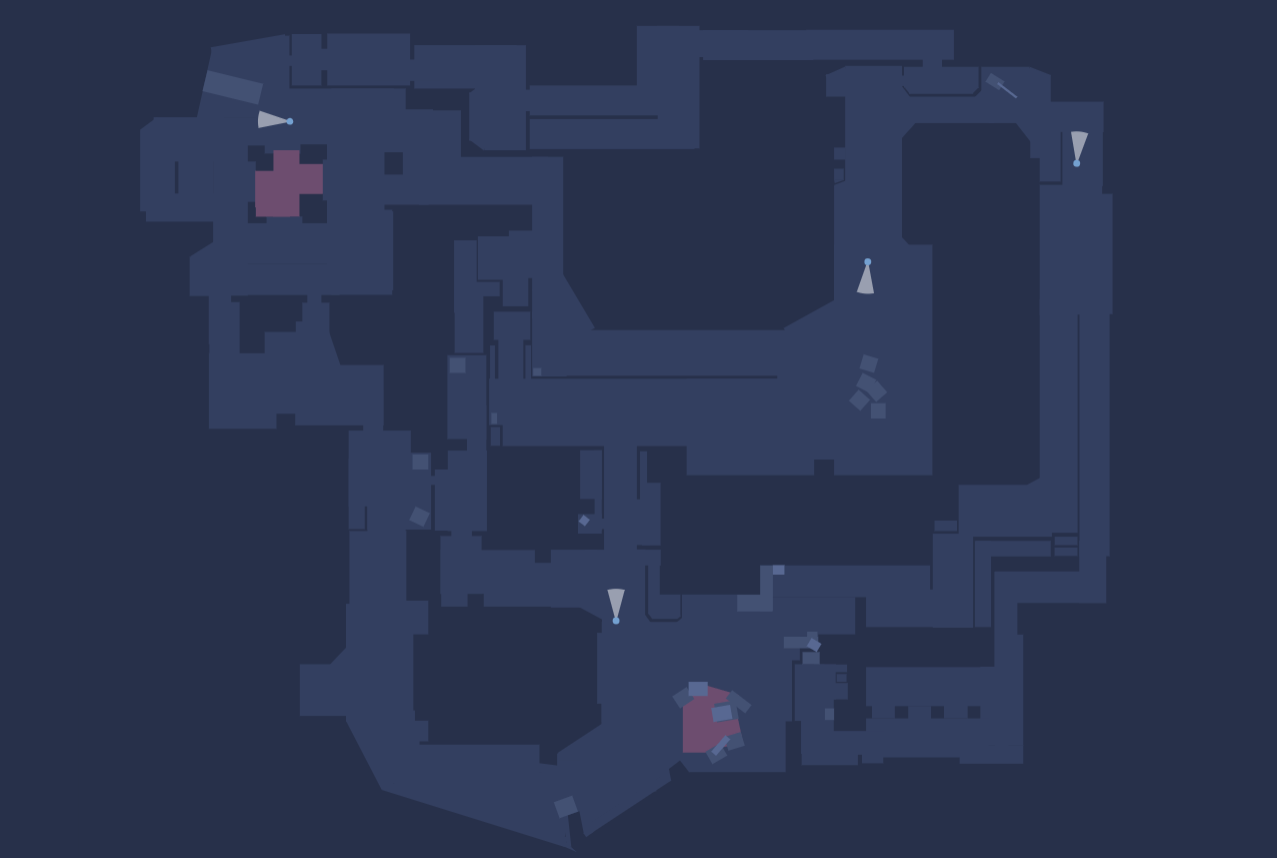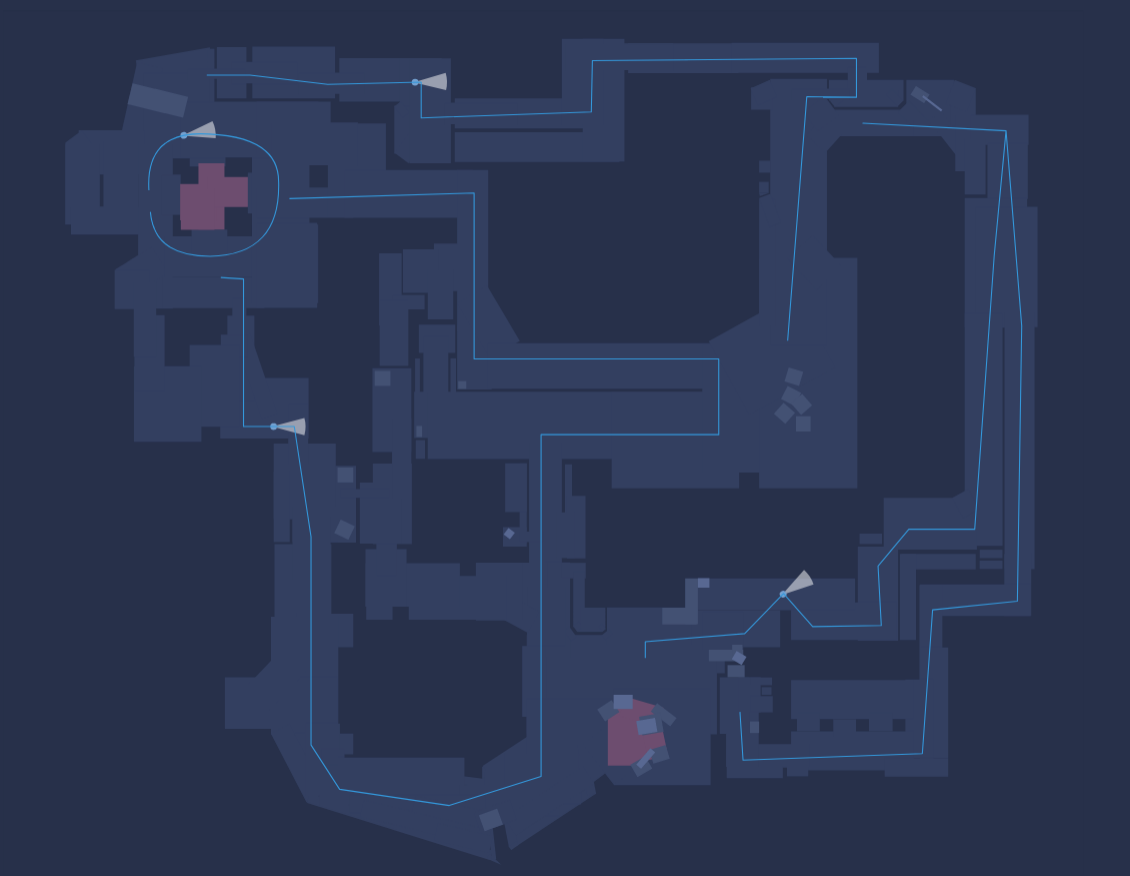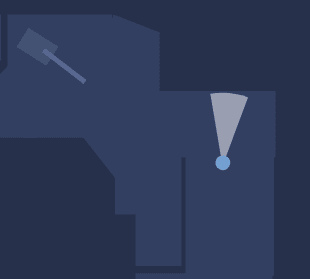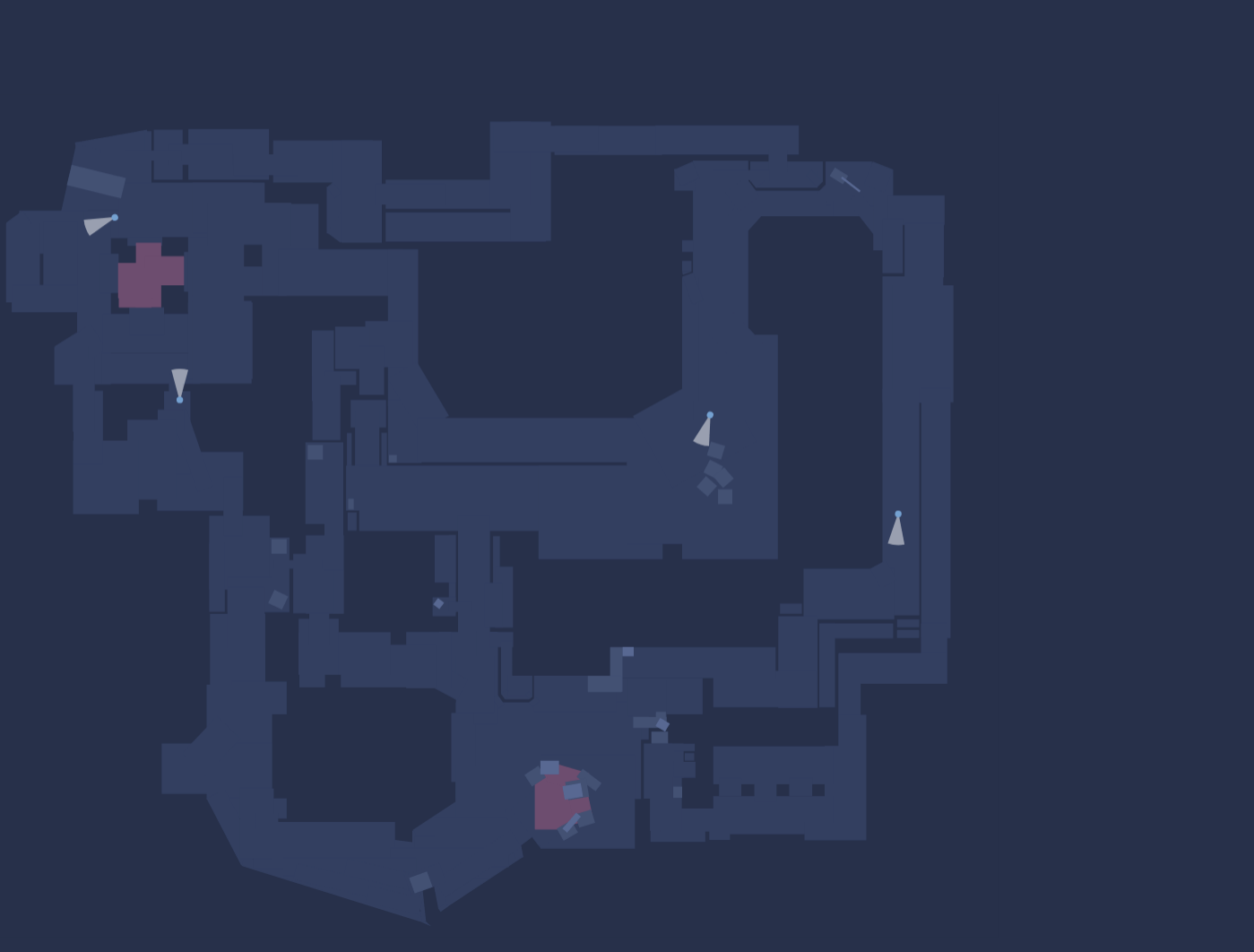## 前言## 代码实现

### 绘制背景色

``````// 画背景
g.save();
g.beginPath();
g.rect(0, 0, 2000, 1600);
g.fillStyle = "rgb(39,48,74)";
g.fill();
g.restore();
});``````

### 加载基础图元``````// 加载人物基础图元
var people = new ht.Node();
// 设置 Tag
people.setTag(id + 'People');
people.setImage('people');
// 设置缩放倍数
people.setScale(10, 10);
people.setPosition(100, 100);
// 设置锚点
people.setAnchor(.5, .2);

### 设置人员属性

• 1: moveTo，占用1个点信息，代表一个新路径的起点
• 2: lineTo，占用1个点信息，代表从上次最后点连接到该点
• 4: bezierCurveTo，占用3个点信息，第一和第二个点作为曲线控制点，第三个点作为曲线结束点
• 5: closePath，不占用点信息，代表本次路径绘制结束，并闭合到路径的起始点``````var people4 = {
id: '437904',
source: {x: 270,y: 352,},
target: {x: 270,y: 352,},
points: [
{x: 262,y: 220,},{x: 380,y: 228,},{x: 509,y: 234,},{x: 509,y: 316,},{x: 513,y: 452,},{x: 382,y: 454,},{x: 278,y: 454,}
],
segments: [1, 3, 3, 3, 3]
};``````

### 获得路径上点和角度

``````var position = graphView.getPercentPosition(data, i);
var angle = graphView.getPercentAngle(data, i) - Math.PI * .5;``````

### 根据路线转角大小控制图元在转角停留时间``````// 转角大小
var rotationAngle = Math.abs((people.getRotation() - angle) % (Math.PI * 2));
// 通过转角大小判断是否需要动画
if (rotationAngle > .2) {
// 判断转角是否大于180度
if (rotationAngle > Math.PI) {
if (people.getRotation() - angle < 0) {
people.setRotation(angle + (Math.PI * 2 - rotationAngle));
} else {
people.setRotation(angle - (Math.PI * 2 - rotationAngle));
}
}``````

### 计算路径总距离，控制行进速度

``````// 计算路径长短
var distance = 0;
for (let i = 0; i + 1 < path.points.length; i++) {
var x = path.points[i + 1].x - path.points[i].x;
var y = path.points[i + 1].y - path.points[i].y;
var pathDistance = Math.sqrt(x * x + y * y);
distance += pathDistance;
}``````

### 增加监听显示标记ID``````var preMoveData, preClickData;
// 增加鼠标移动标记显示
const data = graphView.getDataAt(e);
if (preMoveData === preClickData && (!data || !data.mark)) return;
if (!data || !data.mark) {
preMoveData && preMoveData.mark && preMoveData.mark.s('2d.visible', false);
return;
}
data.mark.s('2d.visible', true);
preMoveData = data;
});
// 增加鼠标点击标记显示
graphView.mi(e => {
if (e.kind === 'clickData' && e.data.mark) {
preClickData && preClickData.mark.s('2d.visible', false);
e.data.mark.s('2d.visible', true);
if (preClickData === e.data) {
e.data.mark.s('2d.visible', false);
preClickData = undefined;
}else {
preClickData = e.data;
}
}
})``````